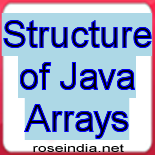# Structure of Java Arrays

Now lets study the structure of Arrays in java. Array is the most widely used data structure in java. It can contain multiple values of the same type.### Structure of Java Arrays

Now lets study the structure of Arrays in java. Array is the most widely used data structure in java. It can contain multiple values of the same type.# Structure of Java Arrays

Now lets study the structure of Arrays in java. Array is the most widely used data structure in java. It can contain multiple values of the same type. Moreover, arrays are always of fixed length i.e. the length of an array cannot be increased or decreased.

Lets have a close look over the structure of Array. Array contains the values which are implicitly  referenced through the index values. So to access the stored values in an array we use indexes. Suppose an array contains "n"  integers. The first element of this array will  be indexed with the "0" value and the last integer will be referenced by "n-1" indexed value.

Presume an array  that contains 12 elements as shown  in the figure. Each element is holding a distinct value. Here the first element is refrenced by a i.e. the first  index value. We have filled the 12 distinct values in the array each referenced as:

a=1

a=2

...

a[n-1]=n

...

a=12

The figure below shows the structure of an Array more precisely.